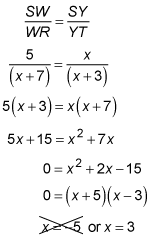##### Geometry: 1,001 Practice Problems For Dummies (+ Free Online Practice)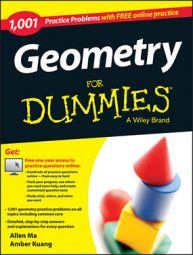When two triangles are similar, this means that their sides are in proportion. The following word problems ask you to use the properties of similar triangles—and a little bit of algebra—to find the solutions.

Similar triangles are different only in size. The corresponding angles still have the same measure.

## Practice questions

1. The lengths of the sides of a triangle are 16, 23, and 31. If the perimeter of a similar triangle is 280, find the length of that triangle's longest side.

2. In triangle RST, W is a point on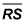and Y is a point on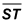such that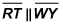If WS = 5, RW = x + 7, YS = x, and TY = x + 3, solve for x.

1. 124

If two triangles are similar, their sides are in proportion. Suppose you call the original triangle A and the new triangle B. The proportion would be as follows: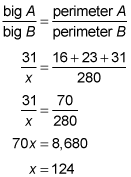2. 3

A line that intersects two sides of a triangle and is parallel to the third side of the triangle creates two similar triangles. If two triangles are similar, their sides are in proportion, and the parts of their sides are also in proportion: